# Quantization in Deep Learning

Deep learning has a growing history of successes, but heavy algorithms running on large graphical processing units are far from ideal. A relatively new family of deep learning methods called quantized neural networks have appeared in answer to this discrepancy. In Leapmind R&D, we are working on quantization methods, among others, for enabling efficient high-performance deep learning computation on small devices.

Neural networks are composed of multiple layers of parameters, each layer transforms the input image, separating and contracting  the feature space, resulting in the separation of input images to their various classes. Perhaps the most notable of deep learning problems are image classification, object detection, and segmentation. Here we focus on image classification, as a basis for the other tasks. For image tasks, convolutional layers are highly valuable, which apply linear transformations to each spatial input patch of the image. Convolutional layers have implicit translational invariance, allowing for a reduction in the number of parameters compared to fully-connected layers.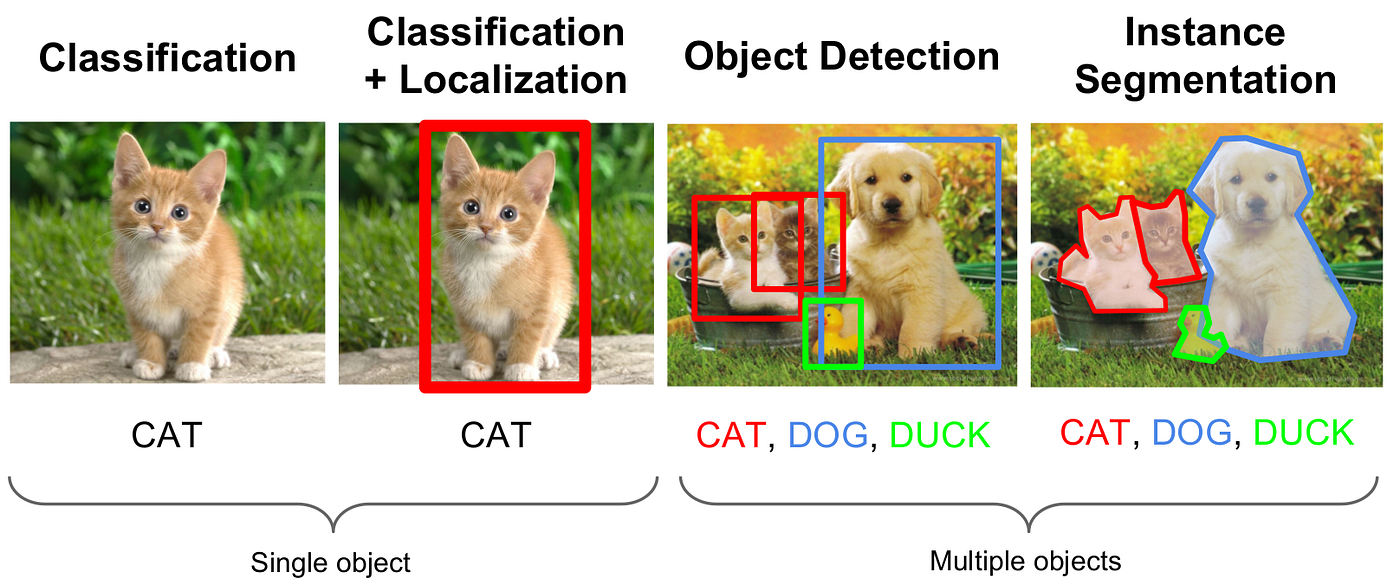from: Standford University 2016 winter lectures CS231n Fei-Fei Li & Andrej Karpathy & Justin Johnson

Deep learning for classification tasks involves training the parameters of a neural network such that the algorithm learns to discern between object classes. This is achieved by feeding many images of labelled data to the neural network, while updating the parameters to increase performance on a smooth objective function. A drawback is that a large number of parameters are used, compared to more traditional algorithms.

Thus enters quantization as a method to bring the neural network to a reasonable size, while also achieving high performance accuracy. This is especially important for on-device applications, where the memory size and number of computations are necessarily limited. Quantization for deep learning is the process of approximating a neural network that uses floating-point numbers by a neural network of low bit width numbers. This dramatically reduces both the memory requirement and computational cost of using neural networks.

# Continuous-discrete learning

The neural network can be quantized after training is finished. However, by far the most effective method for retaining high accuracy is to quantize during training. The oldest example I can find is by A. Choudry et al. , who describe the main idea of neural network parameter quantization during training, which they called continuous-discrete learning. The forward pass of the neural network uses a scheme for rounding float-precision parameters to discrete levels, and in the backwards pass, the float-precision parameters are updated using gradients calculated during the forward pass. That was in 1990 - a time when powerful graphical processing units were not available for training, and most experiments involved small networks that did not enjoy the same success as modern neural networks.

The main concept is illustrated in the figure below - during training there are effectively two networks : float-precision and binary-precision. The binary-precision is updated in the forward pass using the float-precision, and the float-precision is updated in the backward pass using the binary-precision. In this sense, the training is a type of alternating optimization.

Of course, truly binary parameters do not have a derivative. Instead, the binary parameters are treated as if they were float-valued for the purpose of gradient calculation. Another important point is that only the binary-precision network is saved for inference; therefore, the deep learning model size becomes significantly smaller. In general, the size of the model and number of computations during training is not as important - this only happens once. The computation-critical stage is during inference, where the model may be used on small devices, for long durations of time.

Fast forward to 2015, and quantization methods are starting to be used on high performing neural networks. BinaryConnect  quantize the network parameters using a binarizing operation that can be either deterministic or stochastic, which maps float-valued parameters to +1 or -1. The deterministic operation is attractive because of how straightforward it is - simply take the sign of the float-valued parameter. The float-valued parameters are also clipped between +1 and -1, and their update is scaled by the Glorot initialization  to provide extra stability.

Ternary parameter networks are an alternative to binary parameters that allow for higher accuracy, at cost of larger model size. For binary networks, the sign operation minimizes the Frobenius norm of the difference between binary-valued and float-valued parameter matrices. Therefore sign is a natural choice for the binarizing operation. However, it is not so simple for ternary-valued approximation. In particular, the method of F. Li, et al.  define ternarization operation with scaling and threshold that is an effective approximation under the assumption of a parameter distribution which is between Gaussian and uniform. This assumption may not strictly be true, but allows for a relatively simple training scheme.

# Quantized activations

We don’t need to stop with just the parameters of the quantized network - quantizing the inputs to each convolutional layer of the neural network results in massive reduction of necessary computation. Furthermore, the convolution can be carried out in a bitwise manner, which is much more efficient, provided the relevant hardware acceleration is used.

To be able to use quantized inputs for each convolution layer, the activation function is replaced by a quantization function, converting the activations (the network’s internal representation of the input data) to low bit width immediately prior to each convolution. A prime example is the binary network (called BNN) described by M. Courbariaux et al. , who build on their work in , quantizing the activations to binary values -1 and +1 by taking the sign of the full-precision activations. For this binarizing activation, they use gradient-cancelling for activations that were outside the range [-1,1] (to complement their parameter clipping). Another innovation is an approximate shift based batch normalization that uses addition of floating point numbers rather than multiplications, which reduces the necessary computation.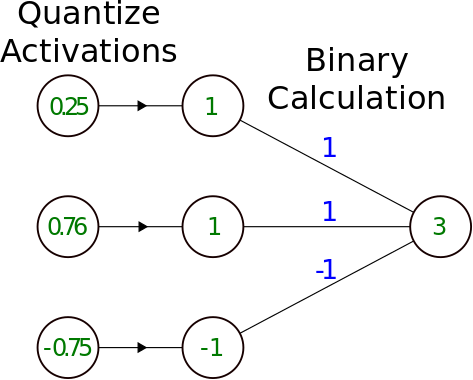Quantization of activations, allowing binary calculations, with integer accumulation. The binary calculation can be convolutional layer or fully-connected layer.

In work by M. Rastegari et al. , the quantized network parameters of a convolutional layer are given by binary -1 and +1 values multiplied by a scaling factor. Scaling factors are calculated per image patch to give an optimal approximation between the binarized and float networks. Since these scaling factors are determined by the input feature maps, they are also calculated during the inference stage, not only during training. This creates an unwanted computational overhead during inference that is analysed by M. Ghasemzadeh et al. . However, as stated in , using fixed scaling factors during inference (only one per output channel of the convolution, rather than per patch), the performance of the network only decreases slightly. This gives a much more attractive option for scaling parameters.

As pointed out in , the first and last layers of a neural network are the most sensitive to quantization and have a relatively small number of computations. Therefore, those two layers can be computed in floating-point precision to achieve higher accuracy without a significant loss in speed. Many of the other quantized networks mentioned here also do not quantize first and last layers.

# Multiple bit width activations

Reducing the bit width of the activations all the way down to binary can harm the accuracy of the neural network. The authors of DoReFa-Net  use an activation quantizer that maps to multiple bits. This can allow higher performance while still achieving great savings in computation. Moreover, they describe a quantization procedure for the gradients with the aim of reducing the amount of computation required for training. However, they find that the gradients require stochastic quantization to be able to get good performance.

In comparison to BNN , the DoReFa-Net authors  do not use parameter clipping or gradient cancelling, and instead use scaling factors (one per layer), so that the quantized parameters are able to match the scale of the float parameters used in training. Their activation quantizer has range between 0 and 1 (again, different to that of BNN ). The general shape of activation quantizers is piecewise constant. For the DoReFa-Net activation quantizer in particular, the quantization levels are equally spaced, and can be seen below.

Other activation quantizers are also possible. For example, Z. Cai et al.  make the argument for different boundaries between quantization levels in order to better handle Gaussian-distributed activations. Also, D. Miyashita et al. advocate for quantization levels at powers of 2, accumulating the activations in the log-domain.

# Expressivity

There has also been promising analysis of the expressive ability of neural networks with quantized parameters. Y. Ding et al.  give upper bounds for the number of layers and quantized parameters necessary to approximate a wide range of functions to arbitrary accuracy. Considering ReLU networks with uniform quantization, the below formulas give upper bounds for the required depth and number of parameters, in the limit of small error.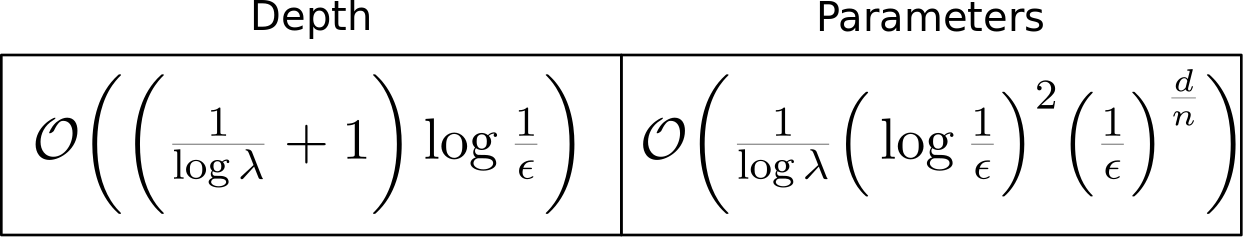Upper bounds of depth and parameters needed for quantized neural network to approximate with arbitrary precision. From: Y. Ding et al. 

In the above formulas, ‘d’ is the input dimension, ’n’ is the number of derivatives that exist for the Sobolev space you would like to approximate functions within, ‘lambda’ is the number of distinct parameters you have in your quantization scheme, and ‘epsilon’ is the error you are willing to allow between the neural network and the function being approximated. Intuitively, smaller error requires larger depth and more parameters. Also, higher-dimensional input and less smooth functions require more parameters to approximate.

Overall, there is a positive outlook for quantization in neural networks, combining high performance with lightweight inference. Here I’ve given a flavour of some modern methods for quantization, which enable deep learning at the edge. The continued creativity in this field is the driver for smarter algorithms that can deliver deep learning to the people.

# References

 S. Mallat, “Understanding deep convolutional networks,” Philosophical
Transactions of the Royal Society A: Mathematical, Physical and Engineering
Sciences, vol. 374, no. 2065, p. 20150203, mar 2016. [Online]. Available:
https://doi.org/10.1098/rsta.2015.0203

 E. Fiesler, A. Choudry, and H. J. Caulfield, “Weight discretization paradigm for optical neural networks,” in Optical Interconnections and Networks, H. Bartelt, Ed. SPIE, aug 1990. [Online]. Available: https://doi.org/10.1117/12.20700

 M. Courbariaux, Y. Bengio, and J. David, “Binaryconnect: Training deep
neural networks with binary weights during propagations,” CoRR, vol.
abs/1511.00363, 2015. [Online]. Available: http://arxiv.org/abs/1511.00363

 X. Glorot and Y. Bengio, “Understanding the difficulty of training deep
feedforward neural networks,” in Proceedings of the Thirteenth International
Conference on Artificial Intelligence and Statistics, ser. Proceedings of
Machine Learning Research, Y. W. Teh and M. Titterington, Eds., vol. 9.
Chia Laguna Resort, Sardinia, Italy: PMLR, 13–15 May 2010, pp. 249–256.
[Online]. Available: http://proceedings.mlr.press/v9/glorot10a.html

 F. Li and B. Liu, “Ternary weight networks,” CoRR, vol. abs/1605.04711, 2016. [Online]. Available: http://arxiv.org/abs/1605.04711

 M. Courbariaux and Y. Bengio, “Binarynet: Training deep neural networks with weights and activations constrained to +1 or -1,” CoRR, vol. abs/1602.02830, 2016. [Online]. Available: http://arxiv.org/abs/1602.02830

 M. Rastegari, V. Ordonez, J. Redmon, and A. Farhadi, “Xnor-net: Imagenet classification using binary convolutional neural networks,” CoRR, vol. abs/1603.05279, 2016. [Online]. Available: http://arxiv.org/abs/1603.05279

 M. Ghasemzadeh, M. Samragh, and F. Koushanfar, “Resbinnet: Residual binary neural network,” CoRR, vol. abs/1711.01243, 2017. [Online]. Available: http://arxiv.org/abs/1711.01243

 S. Zhou, Z. Ni, X. Zhou, H. Wen, Y. Wu, and Y. Zou, “Dorefa-
net: Training low bitwidth convolutional neural networks with low bitwidth gradients,” CoRR, vol. abs/1606.06160, 2016. [Online]. Available: http://arxiv.org/abs/1606.06160

 Z. Cai, X. He, J. Sun, and N. Vasconcelos, “Deep learning with low precision by half-wave gaussian quantization,” CoRR, vol. abs/1702.00953, 2017. [Online]. Available: http://arxiv.org/abs/1702.00953

 D. Miyashita, E. H. Lee, and B. Murmann, “Convolutional neural networks using logarithmic data representation,” CoRR, vol. abs/1603.01025, 2016. [Online]. Available: http://arxiv.org/abs/1603.01025

 Y. Ding, J. Liu, and Y. Shi, “On the universal approximability of quantized relu neural networks,” CoRR, vol. abs/1802.03646, 2018. [Online]. Available: http://arxiv.org/abs/1802.03646

--

--

--

## More from Joel Nicholls

Love podcasts or audiobooks? Learn on the go with our new app.

## Feature Selection using Genetic Algorithm in Python## How to Ace a Machine Learning Career: A Comprehensive Guide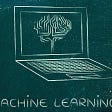## Google & Northwestern U Present Provably Efficient Learning Algorithms for Neural Networks## Weights & Biases — Developer Tools for Machine Learning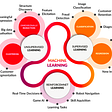## Boltzmann Machine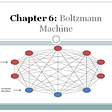## Machine learning basics (part 8): Ensemble learning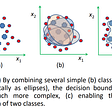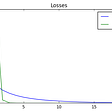## Examining the Transformer Architecture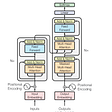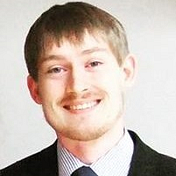## Lights on - PyTorch## An Introductory Guide to Computer Vision## Computer Vision — Where do you even start?